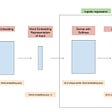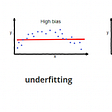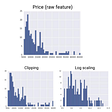Ever wondered about reading a matrix which itself has “confusion” in its name. It is really confusing I know. But don’t worry I am here to solve the confusion & will explain each part of the confusion matrix in detail.

## Introduction to Confusion Matrix

Confusion Matrix is just a way to judge our classification model. For a very basic understanding, each box of confusion matrix just contain the count for each type of prediction by our model which is supported by the confusion matrix.

It is very often used in model evaluation, i.e. to check that how our model performs, or we can say how well our model is predicting the values.

Types of values in Confusion Matrix:

1. True Positive
2. True Negative
3. False Positive (Type 1 Error)
4. False Negative (Type 2 Error)

For a very basic understanding, I have shown here the 2 x 2 confusion matrix.

Always the right predictions will be in left diagonal of the confusion matrix, & confusion matrix will always be a square matrix.

## True Positive

This category has the count of values which are actually True and our model also predicted them to be True.

For Example, if initially the value is True, & our model also stated that the value is True. So, we can conclude that this prediction is right. From the above image you can consider that the predicted value is 1 & true value is also 1.

## True Negative

This category has the count of values which are actually False and our model also predicted them to be False.

For Example, if initially the value is False, & our model also stated that the value is False. So, we can conclude that this prediction is right. From the above image you can consider that the predicted value is 0 & true value is also 0.

## False Positive

They are also knows as Type 1 Errors in the model.

This category has the count of values which are actually False, but our model predicted them to be True.

These are the values which our model predicted wrong.

Examples:

• If initially the value is False, & our model stated that the value is True.
• Let’s say there is a person who is completely fine & it goes for the coronavirus test, but the test report says that the person is affected with coronavirus.

The above example explains the false positive situation.

## False Negative

They are also knows as Type 2 Errors in the model. (Most Dangerous Predictions)

This category has the count of values which are actually True, but our model predicted them to be False.

These are the values which our model predicted wrong.

Examples:

• If initially the value is True, & our model stated that the value is False.
• Let’s say there is a person who is affected with coronavirus & it goes for the coronavirus test, but the test report says that the person is completely fine. So, in this case it will go home happily & there are high chances that can spread the virus to others.

The above example explains the false negative situation, and from the example we can clearly see that how dangerous is the false negative counts.

## Conclusion

Above all the type of values are listed and explained in the best way possible & it is clearly understood that which value count should be the minimum for the model.

Can you guess, which value count I am talking? Yeah, you got it right, the answer is False Negatives.

Hope, the above blog clearly explains the topic `Confusion Matrix` in the best way possible!

--

--

--

## More from Analytics Vidhya

Analytics Vidhya is a community of Analytics and Data Science professionals. We are building the next-gen data science ecosystem https://www.analyticsvidhya.com

## Sync AWS RDS Postgres to Redshift using AWS DMS## Automating Pharmacovigilance: ML and NLP for Detecting Adverse Drug Reactions in Scientific…## From Graduate to Data Scientist: Can You? Can I?## Word2vec from Scratch with NumPy## The 3 Vaccine Dilemmas## Harshit Dawar

Big Data Enthusiast, have a demonstrated history of delivering large and complex projects. Interested in working in the field of AI and Data Science.

## What is a Confusion Matrix ???## Imbalance Datasets in Machine Learning## ML / Bias-Variance, Overfitting-Underfitting## Different Normalization Techniques